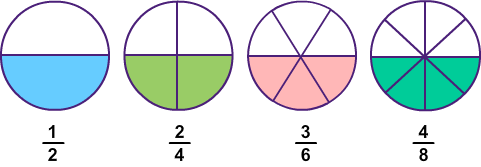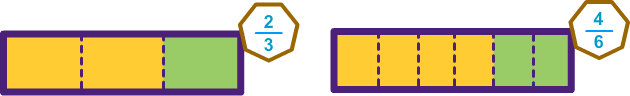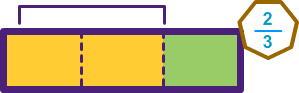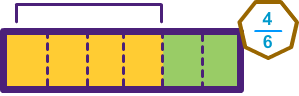# What are Equivalent Fractions?

Print Rate 0 stars Common Core
Lesson size:
Message preview:
Someone you know has shared lesson with you:

To play this lesson, click on the link below:

https://www.turtlediary.com/lesson/equivalent-fractions.html

Hope you have a good experience with this site and recommend to your friends too.

Login to rate activities and track progress.
Login to rate activities and track progress.

Look at the images given below.What do the fractions in the images have in common?

Each fraction in the image represents the same value. These fractions are equivalent.

## Equivalent Fractions

Equivalent fractions are fractions that have different numbers but the same value.

The fractions
1
2
,
2
4
,
3
6
,
4
8
are equivalent since each represents the same value

One way to tell if two fractions are equivalent is to draw a picture.

Let's use a picture to decide if
2
3
and
4
6
are equivalent.

First of all draw 2 boxes and label them:Now divide each box. The first box has a denominator of 3, so split it into 3 sections. The second box has a denominator of 6, split that into 6 sections.Next color these boxes using the numerators. The first fraction has a numerator of 2, so color 2 boxes. The second has a numerator of 4, color 4 boxes.We notice that the same amount of each box is colored, so, these are equivalent fractions.## Arithmetic Procedure of Finding Equivalent Fractions

To find equivalent fractions, multiply or divide the numerator and denominator by the same number.

Let's illustrate through an example.

### Example 1

Are
1
2
and
3
6
equivalent?

To check if two fractions are equivalent multiply or divide by the same number.

We notice that
1
2
is already in the simplest form. So, we can't reduce it further.
Reduce
3
6
by dividing it by 3.

3 ÷ 3
6 ÷ 3
=
1
2

Both fractions reduces to
1
2
, so they are equivalent.

### Example 2

Find two fractions that are equivalent to
2
3
.
To find the equivalent fractions of
2
3
, multiply numerator and denominator by the same number.

Multiply numerator and denominator by 2.

2 x 2
3 x 2
=
4
6

To find another equivalent fraction of
2
3
, multiply numerator and denominator by 3.

2 x 3
3 x 3
=
6
9

So,
4
6
and
6
9
are equivalent fractions of
2
3
.

### Example 3

Find the missing number that makes the fraction equivalent.
3
5
=
---
20

To find the missing number, look at the denominator.

In denominator, 5 is multiplied by 4 to get 20.

So, multiply the numerator 3 by the same number 4 to find the missing number.

3 x 4
5 x 4
=
12
20

3
5
is equivalent to
12
20
.

## What are Equivalent Fractions?

• Equivalent fractions are fractions that have different numbers but the same value.
• We can use pictures to check if two fractions are equivalent.
• To find equivalent fractions, multiply or divide the numerator and denominator by the same number.

## Similar Lessons

Become premium member to get unlimited access.